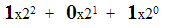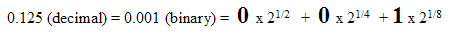# 3. Binary Number

A binary number looks like this:

101110.11001

A binary number only consists of zeroes and ones. Binary fractions are to the right of the binary point.

Example 1- Whole numbers

5 decimal is 101 binary. The relative position of each 1 indicates the exponent of 2 to be used. (Exponent means 'raised to the power of').

Say there is a 1 at position 3, then this represent 2 to the power of 2

A 1 at position 1 represents 2 to the power of zero

So for the number 5 there is a 1 in the third position and a 1 at the 1st position. Reduce these positions by 1 and you end up with a base 2 sequence like this1 times 2 exponent 2 is 4 decimal

0 times 2 exponent 1 is still zero

and 1 times 2 exponent zero is 1

Therefore 4 + 1 = 5. Hence 5 decimal can be represented as 101 binary

Example 2 - Fractions

0.125 decimal (or one-eighth) can be represented as a binary fraction 0.001

This works in a very similar way to whole number except the exponents are now fractions of 2As there is a zero in the exponent half and exponent quarter positions, these add up to zero, but there is a 1 at the exponent one eighths position. So these add up to 0.125 decimal.

You can now combine both methods to represent a 'real number' in binary form.

A real number is a mathematical term that means a number having some decimal parts, like this 1224.99938

### Using a table to convert binary to decimal

Remembering what the various exponent of 2 are can be awkward, so you can quickly form a small table like this. Just double the decimal number at each location above zero and halve the fraction going the other way.

Decimal 64 32 16 8 4 2 1 . 1/2 1/4 1/8 1/16 1/32 1/64 1/128
Binary 1 1 0 1 0 0 0 . 0 1 1 0 0 0 0

So just add the decimal where a 1 is present. Like this

64+32+8+1/4+1/8 = 104.375

Note: the position of the binary point in this format is fixed. This kind of notation is called 'fixed point binary' This format is not often used to represent decimal numbers because there are better ways of doing it. But it does explain the basis of representing a number in binary.

The better ways of representing whole numbers is to use 'integers' and the better way of representing decimal or 'real numbers' is floating point notation. Both these schemes are described in over the next few pages

Challenge see if you can find out one extra fact on this topic that we haven't already told you

Click on this link: Binary number notation